JAJSDS0 September   2017

PRODUCTION DATA.

1. 特長
2. アプリケーション
3. 概要
4. 改訂履歴
5. Pin Configuration and Functions
6. Specifications
7. Detailed Description
1. 7.1 Overview
2. 7.2 Functional Block Diagrams
3. 7.3 Feature Description
4. 7.4 Device Functional Modes
8. Application and Implementation
1. 8.1 Application Information
2. 8.2 Typical Application
3. 8.3 Do's and Don'ts
9. Power Supply Recommendations
10. 10Layout
11. 11デバイスおよびドキュメントのサポート
1. 11.1 デバイス・サポート
1. 11.1.1 開発サポート
2. 11.1.2 デバイスの項目表記
2. 11.2 ドキュメントの更新通知を受け取る方法
3. 11.3 コミュニティ・リソース
4. 11.4 商標
5. 11.5 静電気放電に関する注意事項
6. 11.6 Glossary
12. 12メカニカル、パッケージ、および注文情報

• DQN|4
• DQN|4

## 6 Specifications

### 6.1 Absolute Maximum Ratings

over operating junction temperature range (unless otherwise noted)(1)
MIN MAX UNIT
Voltage(2) IN –0.3 6 V
EN –0.3 6 V
OUT –0.3 6 V
Current (source) OUT Internally limited
Output short-circuit duration Indefinite
Operating junction, TJ –55 150 °C
Storage, Tstg –55 150 °C
Stresses beyond those listed under Absolute Maximum Ratings may cause permanent damage to the device. These are stress ratings only, and functional operation of the device at these or any other conditions beyond those indicated under Recommended Operating Conditions is not implied. Exposure to absolute-maximum-rated conditions for extended periods my affect device reliability.
All voltages are with respect to GND pin.

### 6.2 ESD Ratings

VALUE UNIT
V(ESD) Electrostatic discharge Human body model (HBM) QSS 009-105 (JESD22-A114A)(1) ±2000 V
Charged device model (CDM) QSS 009-147 (JESD22-C101B.01)(2) ±500
JEDEC document JEP155 states that 500-V HBM allows safe manufacturing with a standard ESD control process.
JEDEC document JEP157 states that 250-V CDM allows safe manufacturing with a standard ESD control process.

### 6.3 Recommended Operating Conditions

over operating junction temperature range (unless otherwise noted)
MIN NOM MAX UNIT
VIN Input voltage 2 5.5 V
IOUT Output current 0 200 mA
TJ Operating junction temperature range –40 125 °C

### 6.4 Thermal Information

THERMAL METRIC(1) TLV742P UNIT
DQN (X2SON)
4 PINS
RθJA Junction-to-ambient thermal resistance 180.4 °C/W
RθJC(top) Junction-to-case (top) thermal resistance 152 °C/W
RθJB Junction-to-board thermal resistance 117.2 °C/W
ψJT Junction-to-top characterization parameter 5.1 °C/W
ψJB Junction-to-board characterization parameter 117 °C/W
RθJC(bot) Junction-to-case (bottom) thermal resistance 99.7 °C/W

### 6.5 Electrical Characteristics

at VIN = VOUT(NOM) + 0.5 V or 2 V (whichever is greater); IOUT = 1 mA, VEN = VIN, COUT = 0.47 μF, and TJ = –40°C to +85°C. Typical values are at TJ = 25°C, (unless otherwise noted)
PARAMETER TEST CONDITIONS MIN TYP MAX UNIT
VIN Input voltage range 2 5.5 V
VOUT Output voltage range 0.85 5 V
DC output accuracy 0.5%
VOUT ≥ 0.85 V –1.5% 1.5%
ΔVO(ΔVI) Line regulation 1 5 mV
ΔVO(ΔIO) Load regulation 0 mA ≤ IOUT ≤ 150 mA 10 20 mV
V(DO) Dropout voltage VIN = 0.98 × VOUT(NOM) 2 V < VOUT ≤ 2.4 V IOUT = 30 mA 65 mV
IOUT = 150 mA 325 360 mV
2.4 V < VOUT ≤ 2.8 V IOUT = 30 mA 50 mV
IOUT = 150 mA 250 300 mV
2.8 V < VOUT ≤ 3.3 V IOUT = 30 mA 45 mV
IOUT = 150 mA 220 270 mV
3.3 V < VOUT ≤ 5 V IOUT = 30 mA 40 mV
IOUT = 150 mA 200 250 mV
ICL Output current limit VOUT = 0.9 × VOUT(NOM) 240 300 450 mA
I(GND) Ground pin current IOUT = 0 mA 25 50 µA
I(EN) EN pin current VEN = 5.5 V 0.01 µA
ISHUTDOWN Shutdown current VEN ≤ 0.4 V
2 V ≤ VIN ≤ 4.5 V
1 µA
VIL(EN) EN pin low-level input voltage
(disable device)
0 0.4 V
VIH(EN) EN pin high-level input voltage (enable device) 0.9 VIN V
PSRR Power-supply rejection ratio VIN = 3.3 V
VOUT = 2.8 V
IOUT = 30 mA
f = 100 Hz 70 dB
f = 10 kHz 55
f = 1 MHz 55
Vn Output noise voltage BW = 100 Hz to 100 kHz,
VIN = 2.3 V
VOUT = 1.8 V
IOUT = 10 mA
45 µVRMS
tSTR Startup time(1) COUT = 1 µF
IOUT = 150 mA
100 µs
RPULLDOWN Pulldown resistance
(TLV742P only)
120 Ω
TJ Operating junction temperature –40 125 °C
Start-up time = time from EN assertion to 0.98 × VOUT.

### 6.6 Typical Characteristics

at TJ = –40°C to +85°C, VIN = VOUT(NOM) + 0.5 V or 2 V (whichever is greater), IOUT = 10 mA, VEN = VIN, and COUT = 1 µF Typical values are at TJ = 25°C, (unless otherwise noted)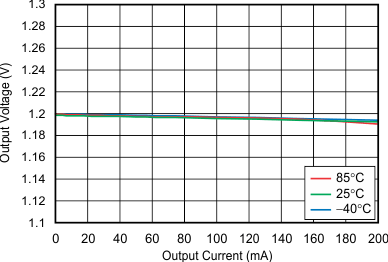VOUT = 1.2 V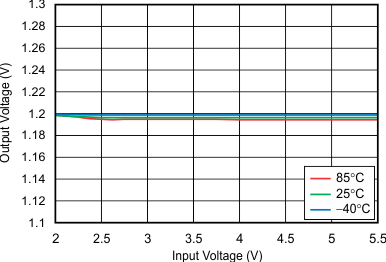VOUT = 1.2 V, IOUT = 10 mA
Figure 3. Line RegulationVOUT = 1.2 V, IOUT = 150 mA
Figure 5. Line Regulation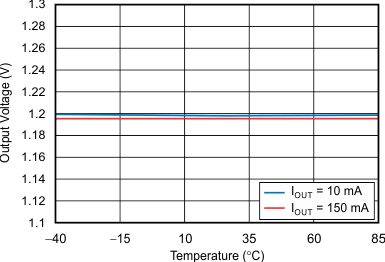VOUT = 1.2 V
Figure 7. Output Voltage vs TemperatureIOUT = 150 mA
Figure 9. Dropout Voltage vs Input Voltage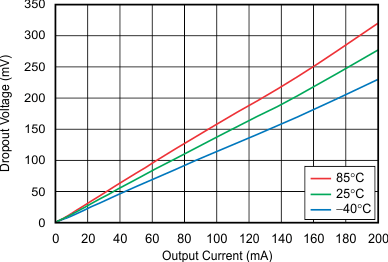VOUT = 2.8 V
Figure 11. Dropout Voltage vs Output Current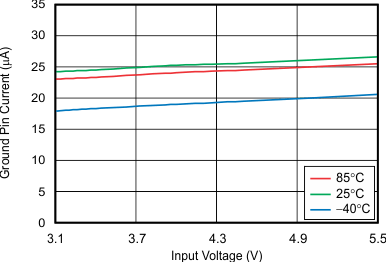VOUT = 2.8 V, IOUT = 0 mA
Figure 13. Ground Pin Current vs Input Voltage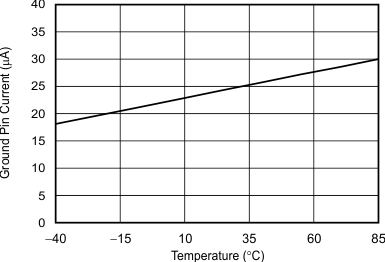VOUT = 2.8 V, IOUT = 0 mA
Figure 15. Ground Pin Current vs Temperature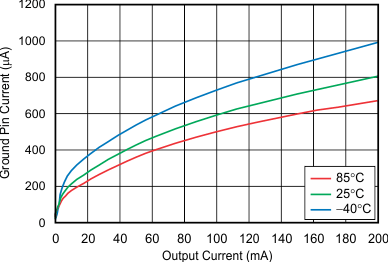VOUT = 2.8 V
Figure 17. Ground Pin Current vs Output Current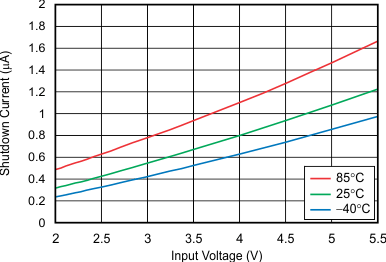VOUT = 2.8 V
Figure 19. Shutdown Current vs Input Voltage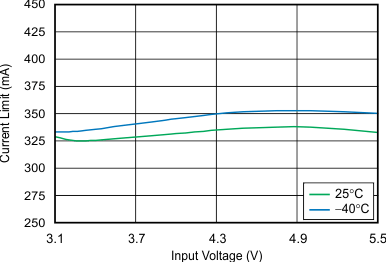VOUT = 2.8 V
Figure 21. Current Limit vs Input Voltage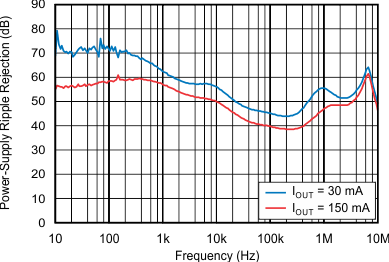VOUT = 2.8 V
Figure 23. Power-Supply Ripple Rejection vs FrequencyVOUT = 2.8 V, IOUT = 150 mA
Figure 25. Power-Supply Ripple Rejection vs Input Voltage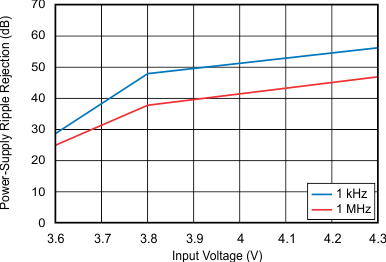VOUT = 3.3 V, IOUT = 150 mA
Figure 27. Power-Supply Ripple Rejection vs Input Voltage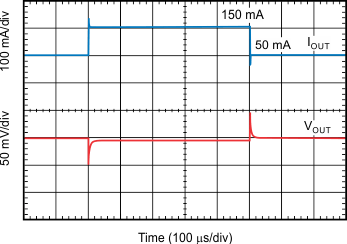VOUT = 1.2 V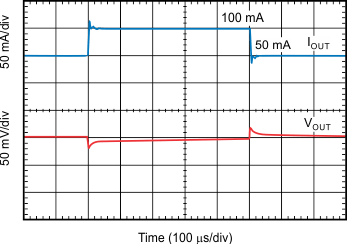VOUT = 2.8 V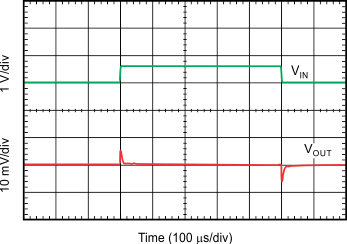VOUT = 1.2 V, IOUT = 150 mA
Figure 33. Line Transient Response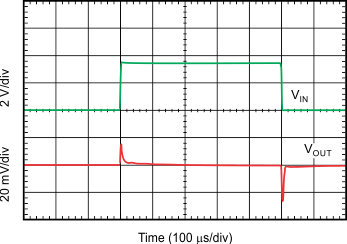VOUT = 1.2 V, IOUT = 150 mA
Figure 35. Line Transient Response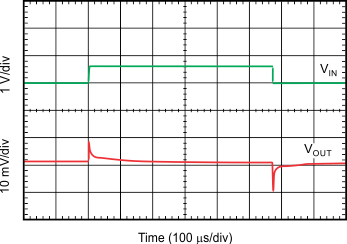VOUT = 2.8 V, IOUT = 150 mA
Figure 37. Line Transient Response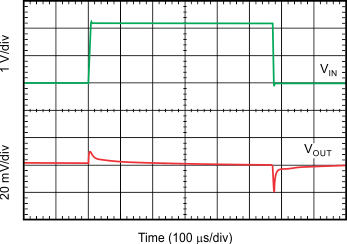VOUT = 2.8 V, IOUT = 200 mA
Figure 39. Line Transient Response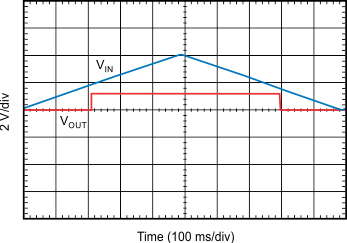VOUT = 1.2 V, IOUT = 30 mA
Figure 41. VIN Ramp Up, Ramp Down Response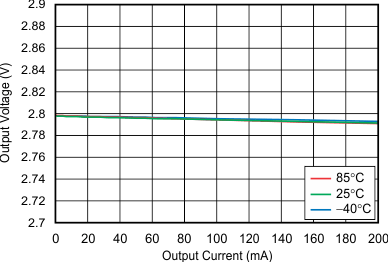VOUT = 2.8 V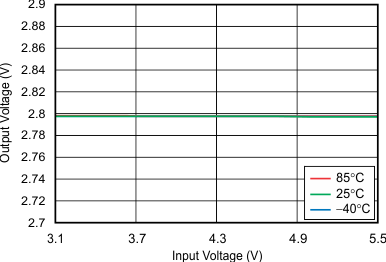VOUT = 2.8 V, IOUT = 10 mA
Figure 4. Line Regulation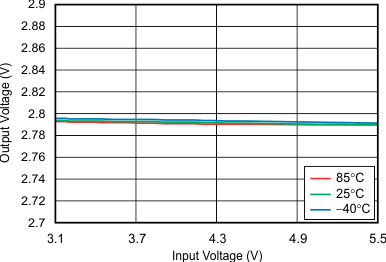VOUT = 2.8 V, IOUT = 150 mA
Figure 6. Line Regulation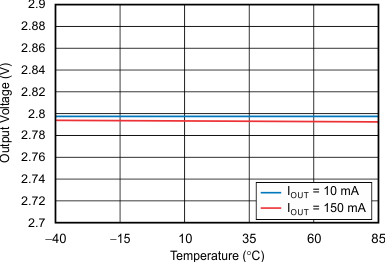VOUT = 2.8 V
Figure 8. Output Voltage vs Temperature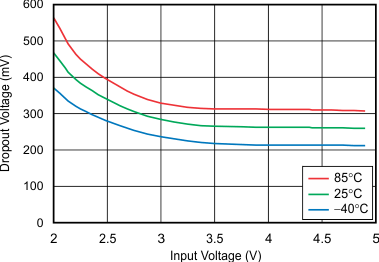IOUT = 200 mA
Figure 10. Dropout Voltage vs Input Voltage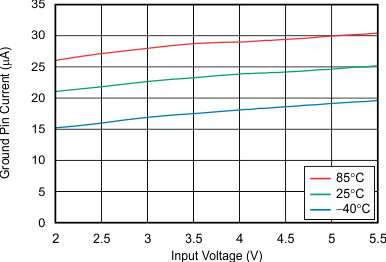VOUT = 1.2 V, IOUT = 0 mA
Figure 12. Ground Pin Current vs Input Voltage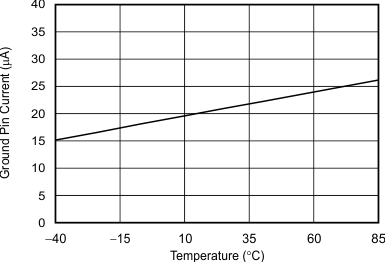VOUT = 1.2 V, IOUT = 0 mA
Figure 14. Ground Pin Current vs Temperature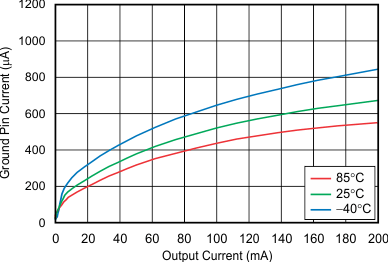VOUT = 1.2 V
Figure 16. Ground Pin Current vs Output Current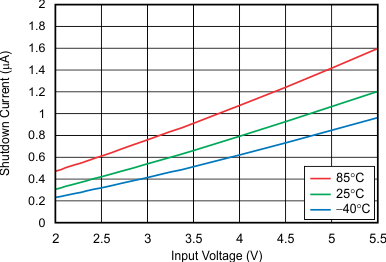VOUT = 1.2 V
Figure 18. Shutdown Current vs Input Voltage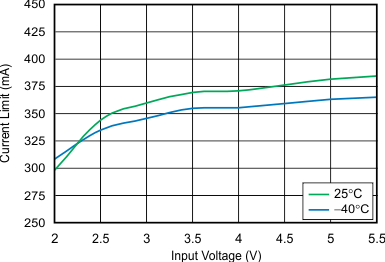VOUT = 1.2 V
Figure 20. Current Limit vs Input Voltage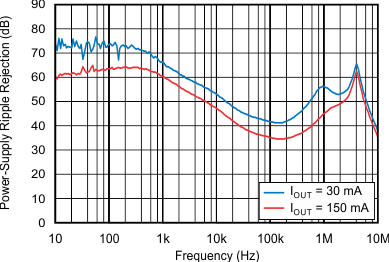VOUT = 1.2 V
Figure 22. Power-Supply Ripple Rejection vs Frequency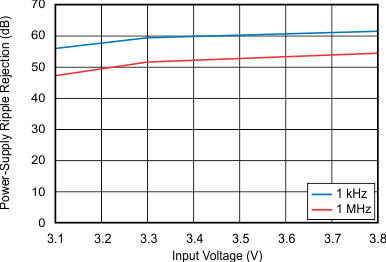VOUT = 2.8 V, IOUT = 30 mA
Figure 24. Power-Supply Ripple Rejection vs Input Voltage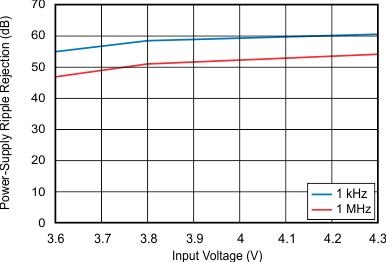VOUT = 3.3 V, IOUT = 30 mA
Figure 26. Power-Supply Ripple Rejection vs Input Voltage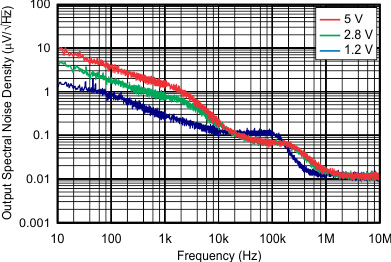Figure 28. Output Spectral Noise Density vs Frequency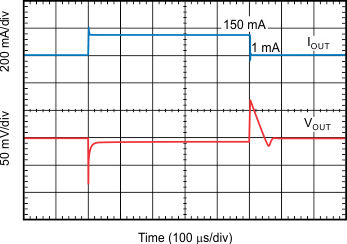VOUT = 1.2 V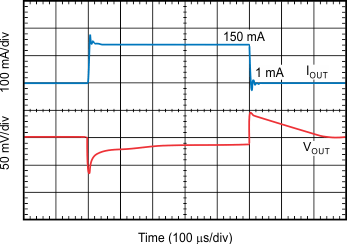VOUT = 2.8 V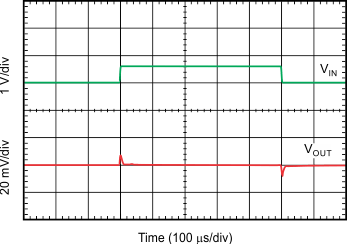VOUT = 1.2 V, IOUT = 200 mA
Figure 34. Line Transient ResponseVOUT = 1.2 V, IOUT = 200 mA
Figure 36. Line Transient Response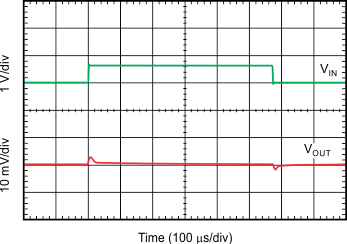VOUT = 2.8 V, IOUT = 200 mA
Figure 38. Line Transient Response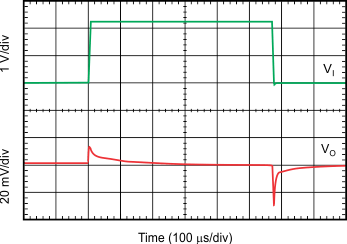VOUT = 2.8 V, IOUT = 200 mA
Figure 40. Line Transient Response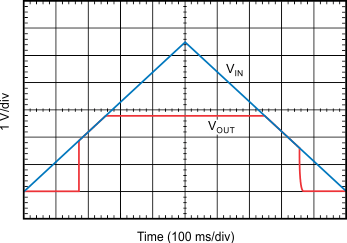VOUT = 2.8 V, IOUT = 30 mA
Figure 42. VIN Ramp Up, Ramp Down Response